Courses

# NCERT Solutions(Part- 4)- Mensuration Class 8 Notes | EduRev

## Class 8 Mathematics by VP Classes

Created by: Full Circle

## Class 8 : NCERT Solutions(Part- 4)- Mensuration Class 8 Notes | EduRev

The document NCERT Solutions(Part- 4)- Mensuration Class 8 Notes | EduRev is a part of the Class 8 Course Class 8 Mathematics by VP Classes.
All you need of Class 8 at this link: Class 8

Surface Area of Cube, Cuboid and Cylinder

Cube: A cube has 6 faces, all of which are congruent squares.

 Total surface area of a cube having length of one side as ‘l’ = 6l2

Remember

1. For a cube, length, breadth and height are all equal.
2. If the length of the edge of a cube is doubled, it surface area increases four times

Cuboid: A cuboid too have 6 faces but they may or may not be equal. All the six faces are rectangular and the opposite faces are identical, Thus, in a cuboid, we get 3 pairs of identical faces.

For a cuboid having length, breadth and height as l, b and h respectively, we have:

 Total surface area = 2(lb + bh + hl)

Note: Area of 4 walls of a room = [perimeter of the room] *height of the room

Cylinder: A cylinder has three surfaces: (i) curved surface, (ii) base surface and (iii) Top surface.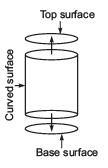Note:

1. The top and bottom (base) surfaces are circular.
2. The top surface and bottom surface are identical.

 Total surface area of a cylinder = 2πr[h + r]

Question: Why is it incorrect to call the solid shown here a cylinder?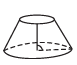Solution: We know that a cylinder has two identical (congruent) circular faces, parallel to each other. Therefore, it is incorrect to call the given solid as a cylinder, because its opposite faces are not congruent.

Question: Find the total surface area of the following cuboids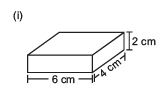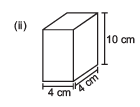Solution: (i) ∵ l = 6 cm, b = 4 cm and h = 2 cm

∴ Total surface area of the cuboids = 2(lb + bh + hl)

= 2(6 * 4 + 4 * 2 + 2 * 6cm2

= 2(24 + 8 + 12) cm2

= 2* 44 cm2 = 88 cm2

(ii) ∵ l = 4 cm, b = 4 cm and h = 10 cm

∴ Total surface area of the cuboid = 2(lb + bh + hl)

= 2(4*4 + 4*10 + 10*4) cm2

= 2(16 + 40 + 40) cm2

= 2*96 cm2 = 192 cm2

Question 1. Can we say that the total surface area of cuboid = lateral surface area + 2 * area of base?

Solution: Yes, or a cuboid,

Total surface area = Lateral surface area + 2 * Area of the base [∵ Opposite faces are congruent]

Question 2. If we interchange the lengths of the base and the height of a cuboid [Fig. (i)] to get another cuboid [Fig. (ii)], will its lateral surface area change?

Solution: Lateral surface area of figure (i) = 2(l + b) * h
Lateral surface area of figure (ii) = 2(h + b) * l

Since, the two results are different.

∴ By changing the position of a cuboid, its lateral surface will change.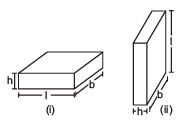Question: Find the surface area of cube A and lateral surface area of cube B.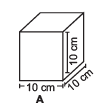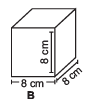Solution: For cube A:

Side (l) = 10 cm

∴ Total surface area of the cube = 6l2

= 6 * (10)2 cm2 = 600 cm2

For cube B:

Side (l) = 8 cm

∴ Total surface area of the cube = 6l2

= 6 * (8)2 cm2

= 6 * 64 cm2 = 256 cm2

Question 1. Two cubes each with side b are joined to form a cuboid figure. What is the surface area of this cuboid? Is it 12b2? Is the surface area of cuboid formed by joining three such cubes, 18b2? Why?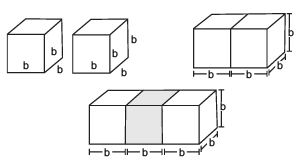Solution: When 2 cubes are joined end to end:

Length (L) = b + b = 2b

Height (H) = b (∵ Height and breadth do not chang.)

∴ Total surface area = 2[LB + BH + HL]

= 2[(2b * b) + (b * b) + (b * 2b)]

= 2[2b2 + b2 + 2b2]

= 2[5b2] = 10b2

∴ The total surface area is not 12b2.

When 3 cubes are joined end to end:

Length (L) = 3b

Height (H) = b (Here too breadth and height do not change.)

∴ Total surface area of the cuboid = 2[LB + BH + HL]

= 2[(3b * b) + (b * b) + (b * 3b)]

= 2[3b2 + b2 + 3b2]

= 2[7b2] = 14b2

∴ The surface area is not 18b2.

Question 2. How will you arrange 12 cubes of equal length to form a cuboid of smallest surface area?

Solution: Case I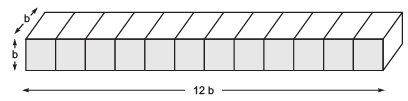Length (L) = 12b

Height (H) = b

∴ Total surface area of the cuboid = 2[LB + BH + HL]

= 2(12b * b) + (b * b) + (b * 12b)]

= 2[12b2 + b2 + 12b2]

= 2[25b2] = 50b2

Case II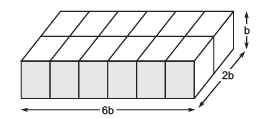Length (L) = 6b

Height (H) = b

∴  Surface area of the cuboid = 2[LB + BH + HL]

= 2[(6b * 2b) + (2b * b) + (b * 6b)]

= 2[12b2 + 2b2 + 6b2]

= 2[20b2] = 40b2

Case III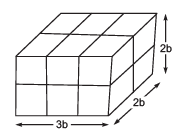Length (L) = 3b

Height (H) = 2b

∴ Surface area of the cuboid = 2(LB + BH + HL)

= 2[(3b * 2b) + (2b * 2b) + (2b * 3b)

= 2[6b+ 4b2 + 6b2]

= 2[16b2] = 32b2

Obviously, the case III arrangement of 12 cubes, has the minimum surface area.

Question 3. After the surface area of cube is painted, the cube is cut into 64 smaller cubes of same dimensions. How many have no face painted? 1 face painted? 2 faces painted? 3 faces painted?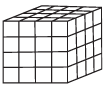Solution: In the given figure, we have:

(a) Number of cubes having no face painted = 16

(b) Number of cubes having 1 face painted = 16

(c) Number of cubes having 2 faces painted = 24

(d) Number of cubes having 3 faces painted = 8

Question: Find total surface area of the following cylinders.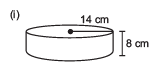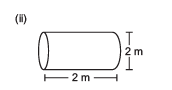Solution:

(i) We have

Height (h) = 8 cm

∴  Total surface area = 2πr(r + h)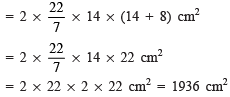(ii) We have r = 2/2 1 m and h = 2 m

∴ Total surface area = 2πr(r + h)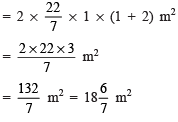Remember

If the cylinder is open then the total surface area is equal to the curved surface area of the cylinder

Offer running on EduRev: Apply code STAYHOME200 to get INR 200 off on our premium plan EduRev Infinity!

93 docs|16 tests

,

,

,

,

,

,

,

,

,

,

,

,

,

,

,

,

,

,

,

,

,

;